# Numbers Line Worksheets

#### What are Number Lines?

In mathematics, number lines are often used to understand and share details about numbers. A number line can be defined as a straight line that has numbers placed at equal segments or intervals along its length. A number line is usually represented horizontally and can be extended infinitely in any direction. Here is an example of a number line: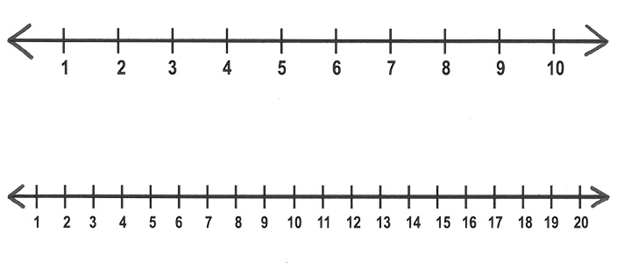As you may have noticed, the numbers increase as you move from left to right and decrease as you move from right to left. Fractions and decimals can also be represented on a number line, as well as both negative and positive integers. Number lines are used because they make it easy to compare numbers. The numbers on the left are smaller as compared to the numbers of the right of the number line. You can use a number line to add, subtract or multiply numbers. To add, we always move right; to subtract, we move left and to multiply, we skip count.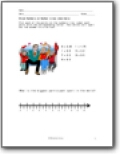###### Mixed Numbers on Number Lines (decimals)

Plot each of the points on the numbers line. Label each point with the corresponding letter. The letters will spell out the answer to a fun fact.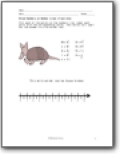###### Mixed Numbers on Number Lines (fractions)

Use the same approach to solve these two riddles: 1. This wild animal can be house broken. 2. On average, how many teeth do bears have?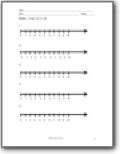###### Blank Number Line Templates

We provide you with a number of sheets that can be used as pre-printed number lines for you to work off of. The intervals of the number lines are provided below.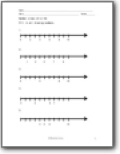###### Intermediate Skills

Fill in all missing numbers across the lines. The interval is noted on each link.